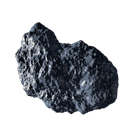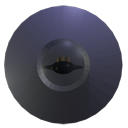DEFCOM1## Loops and Arrays

 Create Asteroid Array Initialise Array Remove Old Asteroid Code Update all Asteroids Draw All AsteroidsCreate an Asteroid Array

What if we want to add more than one asteroid? We can simply add a new asteroid position

Vector2 asteroid2

easy!? but what if we want to add 20, 50, 100???

Well don't know about you, but I'm not going to do that because it's going to take ages!! But there is an easier way. We are going to use an array

Arrays allow us group data together in a table so we can easily work through themasteroid asteroid asteroid asteroid etc...

Lets say asteroid was an actual datatype to create an array of asteroids we would simply say something like

Asteroid[] asteroid = new Asteroid

This would create us an array of 25 asteroids

...but we don't need to do that, we only need the position of 25 asteroids because we are going to use the same image for each one (saves memory and time)

So instead we will do something like this

Vector2[] asteroid = new Vector2

However

we are going to make one change as it will things mucg easier for us later, we are going to add a new variable called numberOfAsteroid

After Creating the UFOPosition delete the asteroid data

```        // Set the coordinates to draw the sprite at.
Vector2 ufoPos = Vector2.Zero;

//create an array of 10 asteroid coordinates (we will use the same image for each one)
static int numberOfAsteroids = 25;
Vector2[] asteroid = new Vector2[numberOfAsteroids];
```

Initialise Array

In the initialise function after the UFO position we are going to set the starting positions of all asteroids

To do this we use a for loop. A for loop reapeats the same set of instruction for a given amount of time.

e.g. for i = 1 to 10 will do something 10 times

We are going to go from 0 to number of asteroids

```            //ufo starting position
ufoPos.Y = screenHeight-128;    //height of screen - hieght of ufo

//Starting Position of all asteroids
for (int i = 0; i < numberOfAsteroids; i++)
{
//position within screen width - ufo width
asteroid[i].X = rand.Next(screenWidth-128);
asteroid[i].Y = 0 - ((i * 250) + 128);
}
```

Remeber to Delete Old Asteroid Code

Update all Asteroids

We will do the same For Loop again in the update function to update the position of all of the asteroids and we now have to check if the ufo has hit any of the asteroids so yes you guessed it.. another For Loop

```            //update all asteroid positions
for (int i = 0; i < numberOfAsteroids; i++)
{
asteroid[i].Y += 5;
if (asteroid[i].Y > screenHeight)
{
Random rand = new Random();
asteroid[i].X = rand.Next(screenWidth - asteroidTex.Width);
asteroid[i].Y = 0 - (numberOfAsteroids * (250-score/10))-128;  //put to top
score += 10;
}
}

//Get Midpoint of ufo
int x = (int)ufoPos.X + (ufoTex.Width / 2);
int y = (int)ufoPos.Y + (ufoTex.Height / 2);

//check if midpoint is inside each asteroid
for (int i = 0; i < numberOfAsteroids; i++)
{
if (x > asteroid[i].X && x < asteroid[i].X + asteroidTex.Width)
{
if (y > asteroid[i].Y && y < asteroid[i].Y + asteroidTex.Height)
{
score = 0; //reset score
}
}
}
```

Draw All Asteroids

And finally in the draw function we need another For Loop to draw all of our asteroids

```spriteBatch.Begin();
spriteBatch.Draw(ufoTex, ufoPos, Color.White);

//draw all asteroids
for (int i = 0; i < numberOfAsteroids; i++)
{
spriteBatch.Draw(asteroidTex, asteroid[i], Color.White);
}

spriteBatch.DrawString(font, "Score: " + score, new Vector2(20, 10), Color.White);

spriteBatch.End();
```

Complete Listings

```public class Game1 : Microsoft.Xna.Framework.Game
{
GraphicsDeviceManager graphics;
SpriteBatch spriteBatch;

//screen size
public int screenHeight;
public int screenWidth;

// This is a texture we can render.
Texture2D ufoTex;
Texture2D asteroidTex;

SpriteFont font;

// Set the coordinates to draw the sprite at.
Vector2 ufoPos = Vector2.Zero;

//create an array of 10 asteroid coordinates (we will use the same image for each one)
static int numberOfAsteroids = 25;
Vector2[] asteroid = new Vector2[numberOfAsteroids];

Random rand = new Random();

int score = 0;

public Game1()
{
graphics = new GraphicsDeviceManager(this);
Content.RootDirectory = "Content";
}

protected override void Initialize()
{
//set Screen size
screenWidth = 800;
screenHeight = 600;

graphics.PreferredBackBufferWidth = screenWidth;
graphics.PreferredBackBufferHeight = screenHeight;

graphics.IsFullScreen = false;
graphics.ApplyChanges();

//ufo starting position
ufoPos.Y = screenHeight-128;    //height of screen - hieght of ufo

//Starting Position of all asteroids
for (int i = 0; i < numberOfAsteroids; i++)
{
//position within screen width - ufo width
asteroid[i].X = rand.Next(screenWidth-128);
asteroid[i].Y = 0 - ((i * 250) + 128);
//float y = asteroid[i].Y;
}

base.Initialize();
}

{
// Create a new SpriteBatch, which can be used to draw textures.
spriteBatch = new SpriteBatch(GraphicsDevice);

}

{
// TODO: Unload any non ContentManager content here
}

protected override void Update(GameTime gameTime)
{
// Allows the game to exit
this.Exit();

//update all asteroid positions
for (int i = 0; i < numberOfAsteroids; i++)
{
asteroid[i].Y += 5;
if (asteroid[i].Y > screenHeight)
{
Random rand = new Random();
asteroid[i].X = rand.Next(screenWidth - asteroidTex.Width);
asteroid[i].Y = 0 - (numberOfAsteroids * (250-score/10))-128;  //put to top
score += 10;
}
}

//Get Midpoint of ufo
int x = (int)ufoPos.X + (ufoTex.Width / 2);
int y = (int)ufoPos.Y + (ufoTex.Height / 2);

//check if midpoint is inside each asteroid
for (int i = 0; i < numberOfAsteroids; i++)
{
if (x > asteroid[i].X && x < asteroid[i].X + asteroidTex.Width)
{
if (y > asteroid[i].Y && y < asteroid[i].Y + asteroidTex.Height)
{
score = 0; //reset score
}
}
}

//update ufo
KeyboardState key = Keyboard.GetState();

if (key.IsKeyDown(Keys.Left) && ufoPos.X > 0)
{
ufoPos.X -= 5;
}
if (key.IsKeyDown(Keys.Right) && ufoPos.X < 800 - ufoTex.Width)
{
ufoPos.X += 5;
}
if (key.IsKeyDown(Keys.Up) && ufoPos.Y > 0)
{
ufoPos.Y -= 5;
}
if (key.IsKeyDown(Keys.Down) && ufoPos.Y < 600 - ufoTex.Height)
{
ufoPos.Y += 5;
}

base.Update(gameTime);
}

protected override void Draw(GameTime gameTime)
{
GraphicsDevice.Clear(Color.Black);

spriteBatch.Begin();
spriteBatch.Draw(ufoTex, ufoPos, Color.White);

//draw all asteroids
for (int i = 0; i < numberOfAsteroids; i++)
{
spriteBatch.Draw(asteroidTex, asteroid[i], Color.White);
}

spriteBatch.DrawString(font, "Score: " + score, new Vector2(20, 10), Color.White);

spriteBatch.End();

base.Draw(gameTime);
}
}
```